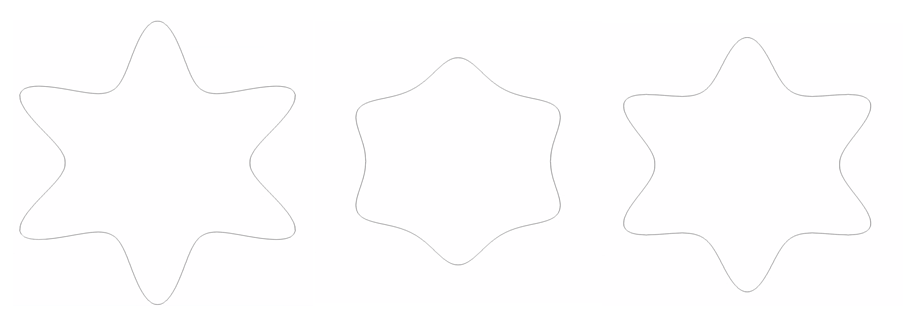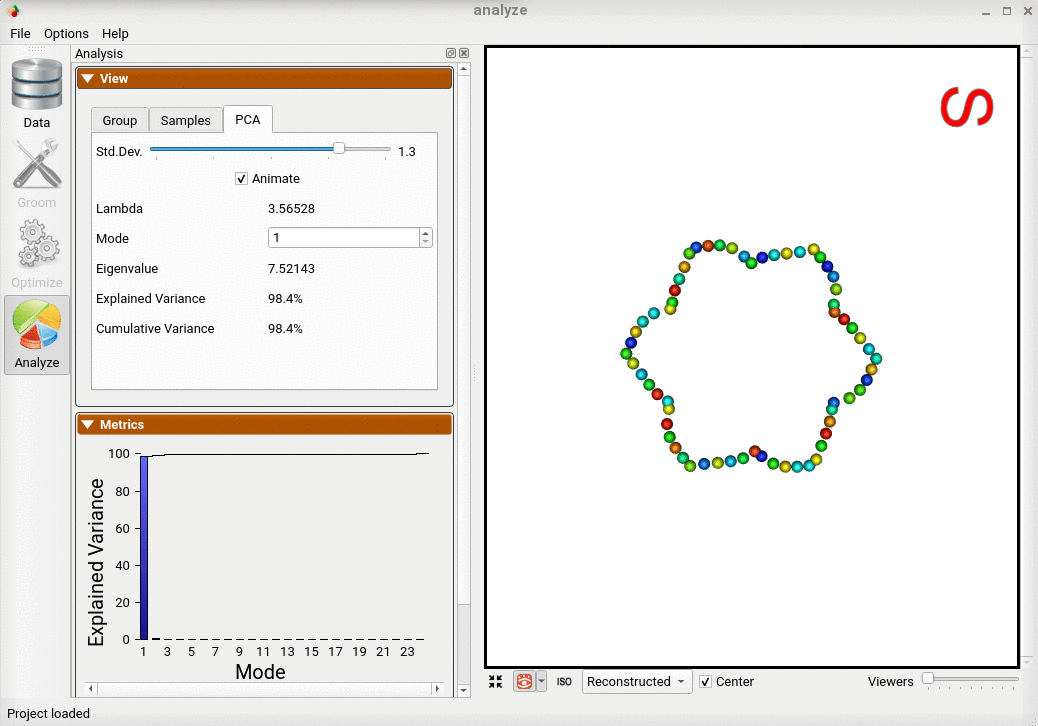# Shape Model for Contour Domains

## What and Where is the Use Case?

This use case demonstrates using ShapeWorks tools to perform the following. - Optimization for N-dimensional contours

The use case is located at: Examples/Python/supershapes_1mode_contour.py

Here is an example of the data:## Running the Use Case

To run the use case, run RunUseCase.py (in Examples/Python/).

$cd /path/to/shapeworks/Examples/Python$ python RunUseCase.py supershapes_1mode_contour


This calls supershapes_1mode_contour.py (in Examples/Python/) to perform the following.

• Loads the supershapes_1mode_contour dataset using a local version if it exists (i.e., previously downloaded); otherwise, the dataset is automatically downloaded from the ShapeWorks Data Portal.
• Optimizes particle distribution (i.e., the shape/correspondence model) by calling optimization functions in OptimizeUtils.py (in Examples/Python/). See Optimizing Shape Model for details about algorithmic parameters for optimizing the shape model.
• Launches ShapeWorks Studio to visualize the use case results (i.e., the optimized shape model and the groomed data) by calling functions in AnalyzeUtils.py (in Examples/Python/).

## Optimizing Shape Model

Below are the default optimization parameters when running this use case. For a description of the optimize tool and its algorithmic parameters, see: How to Optimize Your Shape Model.

{
"number_of_particles" : 64,
"use_normals": 0,
"normal_weight": 0.0,
"checkpointing_interval" : 5000,
"keep_checkpoints" : 0,
"iterations_per_split" : 100,
"optimization_iterations" : 500,
"starting_regularization" : 100,
"ending_regularization" : 1,
"recompute_regularization_interval" : 1,
"domains_per_shape" : 1,
"domain_type" : 'contour',
"relative_weighting" : 5,
"initial_relative_weighting" : 0.1,
"procrustes_interval" : 2,
"procrustes_scaling" : 0,
"save_init_splits" : 0,
"verbosity" : 3,
"use_shape_statistics_after": 4,
}


## Analyzing Shape Model

ShapeWorks Studio visualizes/analyzes the optimized particle-based shape model by visualizing the mean shape, individual shape samples, and the shape modes of variations. For more information, see: How to Analyze Your Shape Model?.